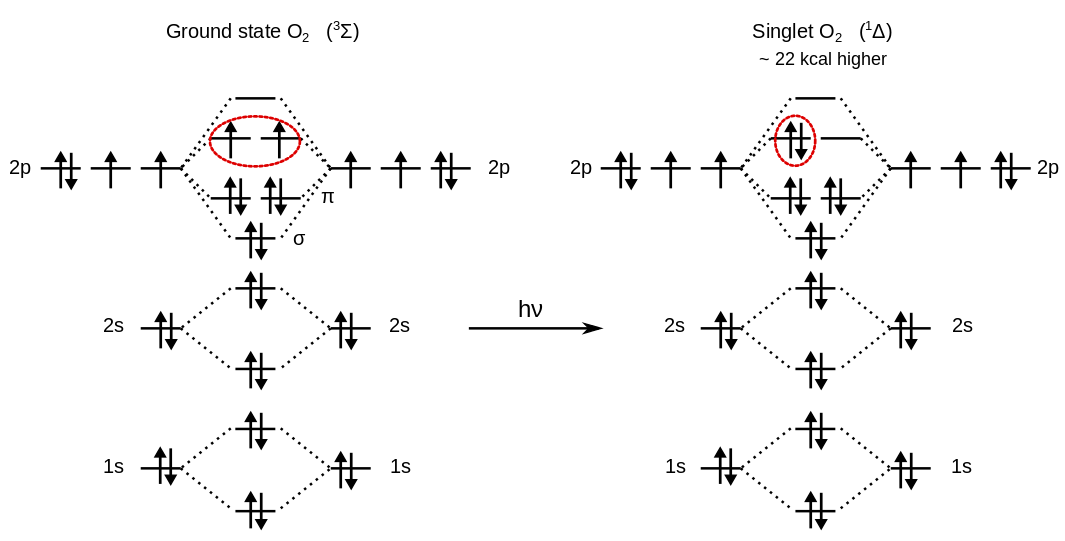#CP2K Open Source Molecular Dynamics

### Site Tools

exercises:2015_ethz_mmm:reaction_energy

# Reaction Energy

In this exercise, you will calculate the reaction energy for the methane combustion reaction: $CH_4 + 2O_2 \rightarrow CO_2 + 2H_2O$

Reaction energy: $\sum E_\text{products} - \sum E_\text{rectants} = \left (2\cdot E_{H_2O} + E_{CO_2} \right) - \left(E_{CH_4} + 2\cdot E_{O_2}\right)$

Ground state oxygen, O$_2$, is a triplet diradical, a property which can explain why liquid oxygen is paramagnetic and attracted to the poles of a magnet.For this reason, to get the energy of the O$_2$ molecule, a LSD calculation is required.

## 1.Step

Run a single point calculation for CH$_4$, using the given input file. Note that the file contains explicit basis sets and potential for all-electron calculations. An explanation of the basis set formats is given here: Basis Sets

CH4.inp
&GLOBAL
PROJECT CH4
RUN_TYPE ENERGY
PRINT_LEVEL MEDIUM
&END GLOBAL

&FORCE_EVAL
METHOD Quickstep              ! Electronic structure method (DFT,...)
&DFT
&POISSON                    ! Solver requested for non periodic calculations
PERIODIC NONE
PSOLVER  WAVELET          ! Type of solver
&END POISSON
&QS                         ! Parameters needed to set up the Quickstep framework
METHOD GAPW               ! Method: gaussian and augmented plane waves
&END QS

&XC                        ! Parametes needed to compute the electronic exchange potential
&XC_FUNCTIONAL NONE      ! No xc functional
&END XC_FUNCTIONAL
&HF                      ! Hartree Fock exchange. In this case is 100% (no fraction specified).
&SCREENING             ! Screening of the electronic repulsion up to the given threshold.
EPS_SCHWARZ 1.0E-10  ! Threshold specification
&END SCREENING
&END HF
&END XC
&END DFT

&SUBSYS
&CELL
ABC 10 10 10
PERIODIC NONE              ! Non periodic calculations. That's why the POISSON section is needed
&END CELL
&TOPOLOGY                    ! Section used to center the atomic coordinates in the given box. Useful for big molecules
&CENTER_COORDINATES
&END
&END
&COORD
C         4.6425962273        5.0574874650        5.2069537560
H         5.7240587065        5.0555482951        5.2189766147
H         4.2766068912        5.8773176685        5.8100567767
H         4.2759350196        4.1226994019        5.6087492584
H         4.2938562590        5.1744089096        4.1899119266
&END COORD
&KIND H                                      ! potential and basis for H
&BASIS
3
1  0  0  3  1
12.25200000          0.02282200
1.86870000          0.15564000
0.41821000          0.48898000
1  0  0  1  1
0.10610000          1.00000000
1  1  1  1  1
1.00000000          1.00000000
&END
POTENTIAL ALL
&POTENTIAL
1    0    0
0.20000000    0
&END
&END KIND
&KIND C                                      ! potential and basis for C
&BASIS
5
1  0  0  6  2
1252.60000000          0.00557360          0.00000000
188.57000000          0.04149600         -0.00027440
42.83900000          0.18263000         -0.00255830
11.81800000          0.46129000         -0.03337500
3.55670000          0.44931000         -0.08730500
0.54258000          0.00000000          0.53415000
1  0  0  1  1
0.16058000          1.00000000
1  1  1  3  1
9.14260000          0.04449900
1.92980000          0.23108000
0.52522000          0.51227000
1  1  1  1  1
0.13608000          1.00000000
1  2  2  1  1
0.80000000          1.00000000
&END
POTENTIAL ALL
&POTENTIAL
4    2    0
0.34883045    0
&END
&END KIND

&END SUBSYS
&END FORCE_EVAL

If the calculation was performed correctly, the total energy of the CH$_4$ molecule is printed in the output file.

  **** **** ******  **  PROGRAM STARTED AT
***** ** ***  *** **   PROGRAM STARTED ON
**    ****   ******    PROGRAM STARTED BY
***** **    ** ** **   PROGRAM PROCESS ID
**** **  *******  **  PROGRAM STARTED IN

.....

ENERGY| Total FORCE_EVAL ( QS ) energy (a.u.):

.....

**** **** ******  **  PROGRAM ENDED AT
***** ** ***  *** **   PROGRAM RAN ON
**    ****   ******    PROGRAM RAN BY
***** **    ** ** **   PROGRAM PROCESS ID
**** **  *******  **  PROGRAM STOPPED IN

## 2.Step

Modify the input in order to perform the same calculation for:

• H$_2$O
• CO$_2$
• O$_2$ triplet
Atomic coordinates for all the molecules, POTENTIAL and BASIS SET for KIND O are given at the end of the exercise.
Remember that the O2 ground state is a triplet state, with non paired electrons. MULTIPLICITY=2S+1=3.

For O2 triplet, the LSD and MULTIPLICITY keywords are needed in the DFT section:

  METHOD Quickstep
&DFT
LSD                           ! Requests a spin-polarized calculation for unpaired electrons
MULTIPLICITY 3                ! Multiplicity = 2S+1 (S= total spin momentum)
...

Another example can be found here Basis Sets

## 3.Step

At the end, you should get a table like:

Species Total Energy
CH$_4$
O$_2$
H$_2$O
CO$_2$

Now you can compute the overall reaction energy.

## Questions

1. What are the total energies of O$_2$, H$_2$O, CO$_2$, and CH$_4$?
2. What is the overall reaction energy of the CH$_4$ combustion?
3. (Optional) What is the total energy difference between the O$_2$ singlet and triplet state?

## Appendix

### Basis Set for Oxygen

#O  pc-1
5
1  0  0  6  2
2306.70000000          0.00539400          0.00000000
347.15000000          0.04024800         -0.00031692
78.89000000          0.17921000         -0.00259440
21.87600000          0.45978000         -0.03624100
6.66460000          0.45234000         -0.08779000
1.06690000          0.00000000          0.53320000
1  0  0  1  1
0.30700000          1.00000000
1  1  1  3  1
17.02200000          0.04891900
3.68380000          0.24962000
0.99234000          0.51347000
1  1  1  1  1
0.24487000          1.00000000
1  2  2  1  1
1.00000000          1.00000000

### Potential for Oxygen

#O ALLELECTRON ALL
4    4    0
0.24762086    0

### Coordinates for O$_2$

O         4.4720538104        4.7584649515        4.9999999998
O         5.5279461896        5.2415350485        4.9999999995

### Coordinates for CO$_2$

  C         4.9999776408        4.9999662056        4.9999894728
O         5.6486993295        5.9339540261        5.0004691016
O         4.3512530072        4.0659797648        4.9995464311

### Coordinates for H$_2$O

  O         4.6926974603        4.7525411835        4.6307067609
H         5.6350172910        4.8022721035        4.7052454388
H         4.3528571397        5.2445222023        5.3644975249